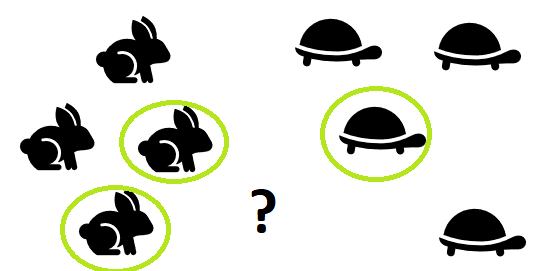# Machine Learning — K-Nearest Neighbors(K-NN)Statistic How To (2019)
1. Definition

The K-Nearest Neighbors (K-NN) algorithm is one of the most fundamental and simple classification scheme which can be used for both classification and regression problems. However, the algorithm is mostly used for classification predictive problems in industry. ‘Feature similarity’ is used to predict the values of new data points which we wish to classify. The ‘feature similarity’ suggests that the new data point will be assigned a value which depends on the ‘closeness’ of it with the data points in the training set.

2. The Algorithm

Suppose now we have a dataset, we would like to implement K-NN algorithm, we have already known that the algorithm depends on the ‘closeness’ of the test sample and the specified training samples, thus, we should find a way to measure the distance between them. There are several distance metrics could be used to calculate the distance, the most commonly used method is called Euclidean distance. The function is defined as follows:

Other distance measure functions includes the Manhattan and the Minkowski.

After we calculated the distance between the new data point and all other points, we could sort the distance values in ascending order. If we take the first row from the top which is the smallest distance value, that will be 1-Nearest Neighbor, then we will classify the new data point in the same category as its ‘neareast neighbor’.

Instead of taking one value, we can extend the idea to a K-NN rule by taking the first K (K can be any integer) rows from the sorted array. Then, we will classify the new data point based on the majority of the K-NN votes in the list.

The choice of K is somewhat arbitrary, however, K can be adjusted based on the size of the dataset and the accuracy of predicting. Some experimentation of different value of K is needed in order to get the best value of K which could minimize the misclassification rate in the validation data. If the value of K is too small, the new data point which we need to classify will be very sensitive to the classification of the closest. A larger value of K will reduce the variability, however, if the value of K is too large, it will induce bias into the classification decisions. Usually, the value of K we use will be between 1 and 20.

To sum it up, In order to implement K-NN algorithm, we could follow the steps below:

• First, we got our dataset, load the train data and test data.
• Choose a specified value of K, then for each data point in the test data, we measure the distance between the point and all other data points in train data.
• Based on the distance values and the value of K which we chose, we classify the new data point based on the majority of the votes in the list.
• Check the predicting accruacy of different values of K, then we pick the K with highest accruacy.

3. Example

Now let us implement the K-NN algorithm on the dataset in Python. The Iris dataset is used in this case.

The Iris dataset could be download here: https://archive.ics.uci.edu/ml/machine-learning-databases/iris/iris.data

We first take a look at the dataset:

The dataset has four features (X) and the column ‘Class’ is our y.

The next step we need to normalize the data, this procedure is important in order to ensure that equal weight are given to each variable. Then we split the data into train set and test set with the help of ‘train_test_split’.

After the preparation is done, we could implement the algorithm, we will make the value of K equal to 4 first to get the model, and we will also calculate the accruacy in this case.

For the value of K equals to 4, the K-NN gives us 91.67% accuracy for the test set. The predicting result is not bad, let us see how the other values of K performs. We would range the value of K from 1 to 9.

As we can see, when the value of K equls to 8, the K-NN gives us the highest accuracy for the test set of 98.33%.

Reference

Evans, J. (2017) Business Analytics. England: Pearson.

Madhu Sanjeevi (2017) ‘Chapter 5 : K-nearest neighbors algorithm with code from scratch.’

Tutorialspoint (2020) ‘KNN Algorithm — Finding Nearest Neighbors’. https://www.tutorialspoint.com/machine_learning_with_python/machine_learning_with_python_knn_algorithm_finding_nearest_neighbors.htm